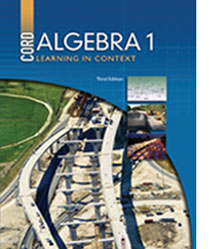# Algebra 1 - 3rd Edition

## Chapter 12: Rational Functions

Some links are repeated for use with more than one lesson.

### 12.1 Rational Expressions

http://www.sparknotes.com/math/algebra2/rationalexpressions/
This site contains links to pages about ration expression terms and problems, as well as adding, subtracting, multiplying, and dividing ration expressions.

http://www.analyzemath.com/Rational_expressions/Rational_express.html

### 12.2 Simplifying Rational Expressions

http://www.purplemath.com/modules/rtnldefs2.htm

http://www.algebralab.org/lessons/lesson.aspx?file=Algebra_rational_simplifying.xml
This site has notes and examples on how to simplify rational expressions.

### 12.3 Multiplying and Dividing Rational Expressions

http://www.purplemath.com/modules/rtnlmult.htm

http://www.purplemath.com/modules/rtnlmult2.htm